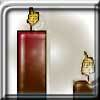#### You may also like### Burning Down

One night two candles were lit. Can you work out how long each candle was originally?### Percentage Unchanged

If the base of a rectangle is increased by 10% and the area is unchanged, by what percentage is the width decreased by ?### Digit Sum

What is the sum of all the digits in all the integers from one to one million?

# Cancelling Fractions

##### Age 14 to 16 ShortChallenge Level

Find a fraction $\frac{m}{n}$ ($m \neq n$) such that all of the fractions

$$\frac{m}{n},\ \frac{m+1}{n+1},\ \frac{m+2}{n+2},\ \frac{m+3}{n+3},\ \frac{m+4}{n+4},\ \frac{m+5}{n+5}$$

can be simplified by cancelling.

This problem is taken from the UKMT Mathematical Challenges.
You can find more short problems, arranged by curriculum topic, in our short problems collection.